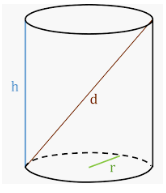Question 2

# What is the farthest distance between two points on a cylinder of: HEIGHT 8 and RADIUS 8 ?

SolutionHeight of cylinder = 8 units and radius = 8 units

=> Farthest distance = hypotenuse = $$\sqrt{(8)^2+(16)^2}$$

= $$8\sqrt{5}$$ units

=> Ans - (A)

### View Video Solution

• All Quant Formulas and shortcuts PDF
• 40+ previous papers with solutions PDF

2 years ago

So many of the gk solutions are wrong in the test!!!!

##### shubhi dubey

2 years, 1 month ago

##### Kushagra Bansal

2 years, 2 months ago